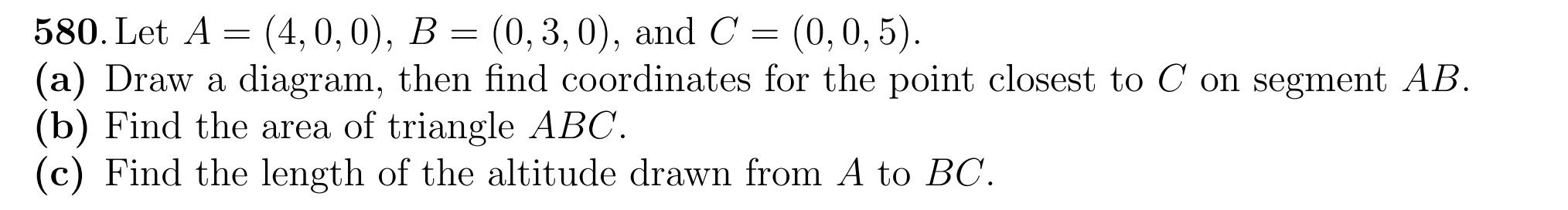# (Solved): 580. Let A = (4, 0, 0), B = (0, 3, 0), and C = (0, 0, 5). (a) Draw a diagram, then find coordinates ...

580. Let A = (4, 0, 0), B = (0, 3, 0), and C = (0, 0, 5). (a) Draw a diagram, then find coordinates for the point closest to C on segment AB. (b) Find the area of triangle ABC. (c) Find the length of the altitude drawn fromA to BC.

580. Let , and (a) Draw a diagram, then find coordinates for the point closest to on segment . (b) Find the area of triangle . (c) Find the length of the altitude drawn from to .

We have an Answer from Expert